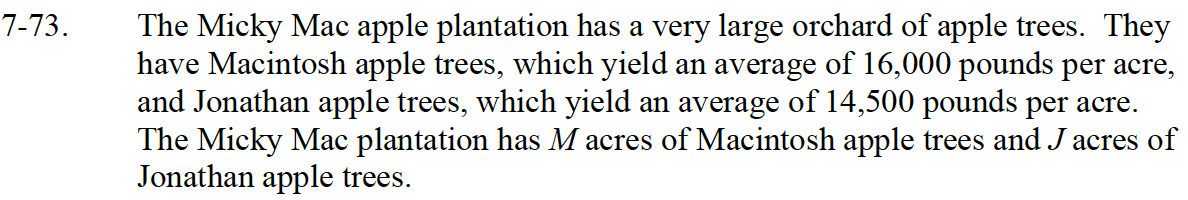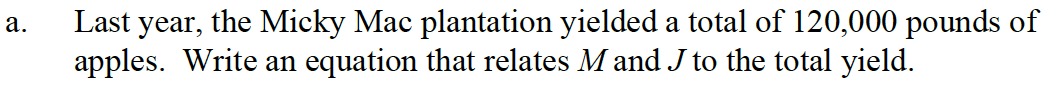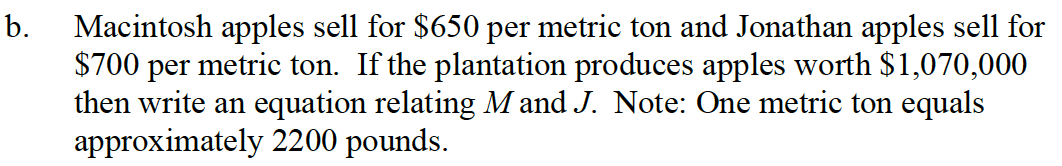Home > CC4 > Chapter 7 > Lesson 7.1.6 > Problem7-73

7-73.
1. The Micky Mac apple plantation has a very large orchard of apple trees. They have Macintosh apple trees, which yield an average of 16,000 pounds per acre, and Jonathan apple trees, which yield an average of 14,500 pounds per acre. The Micky Mac plantation has M acres of Macintosh apple trees and J acres of Jonathan apple trees. Homework Help ✎

1. Last year, the Micky Mac plantation yielded a total of 120,000 pounds of apples. Write an equation that relates M and J to the total yield.

2. Macintosh apples sell for $650 per metric ton and Jonathan apples sell for$700 per metric ton. If the plantation produces apples worth $1,070,000 then write an equation relating M and J. Note: One metric ton equals approximately 2200 pounds.How many pounds of Macintosh apples can be produced in M acres? How many pounds of Jonathan apples can be produced in J acres? How does this relate to 120,000 lbs in the problem? 16000 M + 14500 J = 120000If an orchard of Macintosh apples yields 16000 lbs of apples an acre, how many tons is it? If an orchard of Jonathan apples yields 14500 lbs of apples an acre, how many tons is it? Remember 2200 lbs = 1 metric ton.$650(7.27 M) + $700 (6.59 J) =$1070000 or $4725.50 M +$4613.00 J = \$1070000